Publication date: 03/23/2021

## Nonlinear Path

To model nonlinear degradation paths, select Degradation Path Style > Nonlinear Path from the Degradation Data Analysis red triangle menu. This is useful if a degradation path cannot be linearized using transformations, or if you have a custom nonlinear model that you want to fit to the data.

To facilitate explaining the Nonlinear Path Model Specification, open the Device B.jmp data table. The data consists of power decrease measurements taken on 34 units, across four levels of temperature. Follow these steps:

1. Select Help > Sample Data Library and open Reliability/Device B.jmp.

2. Select Analyze > Reliability and Survival > Degradation.

3. Select Power Drop and click Y, Response.

4. Select Hours and click Time.

5. Select Degrees C and click X.

The temperature setting is the accelerating factor in the experiment.

6. Select Device and click Label, System ID.

7. Click OK.

The initial overlay plot of the data appears.

Figure 7.7 Device B Overlay Plot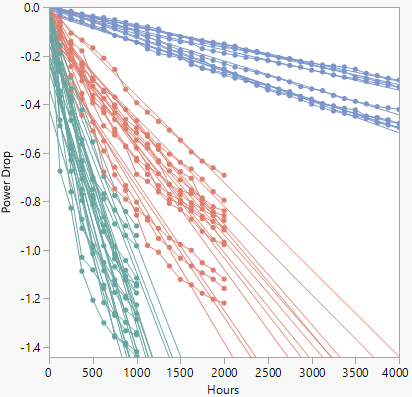The degradation paths appear linear for the first several hundred hours, but then start to curve. To fit a nonlinear model, select Degradation Path Style > Nonlinear Path from the Degradation Data Analysis red triangle menu to show the Nonlinear Path Model Specification outline (Figure 7.8).

Note: To view the Edit button displayed in Figure 7.8, you must select the interactive formula editor preference (File > Preferences > Platforms > Degradation > Use Interactive Formula Editor) before launching the Degradation platform.

Figure 7.8 Initial Nonlinear Model Specification Outline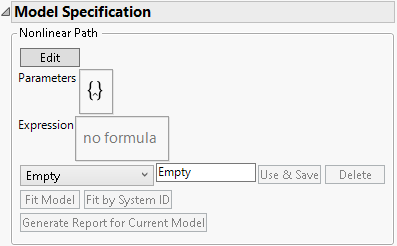The first step to create a model is to select one of the options on the menu initially labeled Empty:

For more information about Reaction Rate models, see Reaction Rate Models.

For more information about Constant Rate models, see Constant Rate Models.

For more information about using a Prediction Column, see Prediction Columns.

### Reaction Rate Models

The Reaction Rate options are applicable when the degradation occurs from a single chemical reaction, and the reaction rate is a function of temperature only. Select Reaction Rate or Reaction Rate Type 1 from the menu shown in Figure 7.8. Although similar to the Reaction Rate model, the Reaction Rate Type 1 model contains an offset term that changes the basic assumption concerning the response value’s sign.

The Setup window prompts you to select the temperature scale, and the baseline temperature. The baseline temperature is used to generate initial estimates of parameter values. The baseline temperature should be representative of the temperatures used in the study.

For this example, select Reaction Rate and then select Celsius as the Temperature Unit. Click OK to return to the report. For more information about all the features for Model Specification, see Model Specification Details.

### Constant Rate Models

The Constant Rate option is for modeling degradation paths that are linear with respect to time (or linear with respect to time after transforming the response or time). The reaction rate is a function of temperature only.

Select Constant Rate from the menu shown in Figure 7.8. The Constant Rate Model Settings window prompts you to enter transformations for the Path, Rate, and Time.

Figure 7.9 Constant Rate Transformation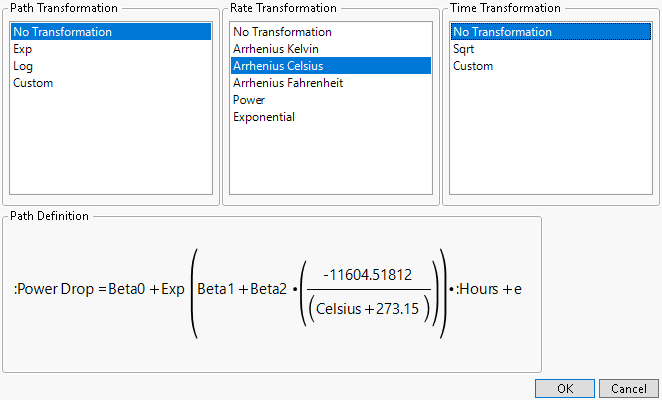Once a selection is made for each transformation, the associated formula appears in the lower left corner as shown in Figure 7.9.

After all selections are made, click OK to return to the report. For more information about all the features for Model Specification, see Model Specification Details.

### Prediction Columns

The Prediction Column option enables you to use a custom model that is stored as a formula in a data table column. The easiest approach is to create the formula column before launching the Degradation platform. You can also create the formula column from within the Degradation platform if you want to use one of the built-in models in the Nonlinear Model Library.

For more information about how to create a custom model and store it as a column formula, see Fit a Custom Model or Nonlinear Regression in Predictive and Specialized Modeling.

Select Prediction Column from the list that appears beneath the Expression area in Figure 7.8. The Model Specification outline changes to prompt you to select the column that contains the model.

Figure 7.10 Column Selection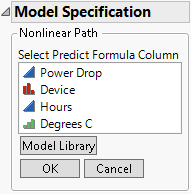At this point, do one of three things:

If the model that you want to use already exists as a formula in a column of the data table, select the corresponding column here, and then click OK. You are returned to the Nonlinear Path Model Specification. For more information about all the features for that specification, see Model Specification Details.

If the model that you want to use does not already exist in the data table, you can click the Model Library button to use one of the built-in models. For more information about using the Model Library button, see Model Library or Nonlinear Regression in Predictive and Specialized Modeling. After the model is created, select Redo > Redo Analysis from the Degradation Data Analysis red triangle menu. Then, return to the column selection shown in Figure 7.10. Select the column that contains the model, and then click OK. You are returned to the Nonlinear Path Model Specification. For more information about all the features for that specification, see Model Specification Details.

If the model that you want to use is not in the data table, and you do not want to use one of the built-in models, then you are not ready to use this model specification. First, create the model, relaunch the Degradation platform, and then return to the column selection (Figure 7.10). Select the column containing the model, and then click OK. You are returned to the Nonlinear Path Model Specification. For more information about all the features for that specification, see Model Specification Details.

### Model Specification Details

After you select one of the model types and supply the required information, you are returned to the Nonlinear Path Model Specification window.

Note: To view the Edit button displayed in Figure 7.11, you must select the interactive formula editor preference (File > Preferences > Platforms > Degradation > Use Interactive Formula Editor) before launching the Degradation platform.

A model is now shown in the script box that uses the Parameter statement. Initial values for the parameters are estimated from the data. For more information about creating models that use parameters, see Fit a Custom Model or Nonlinear Regression in Predictive and Specialized Modeling.

If desired, enter a name in the text box to name the model. For this example, use the name “Device RR”. After that, click the Use & Save button to enter the model and activate the other buttons and features. Figure 7.11 shows the Model Specification window after clicking the Use & Save button.

Figure 7.11 Model Specification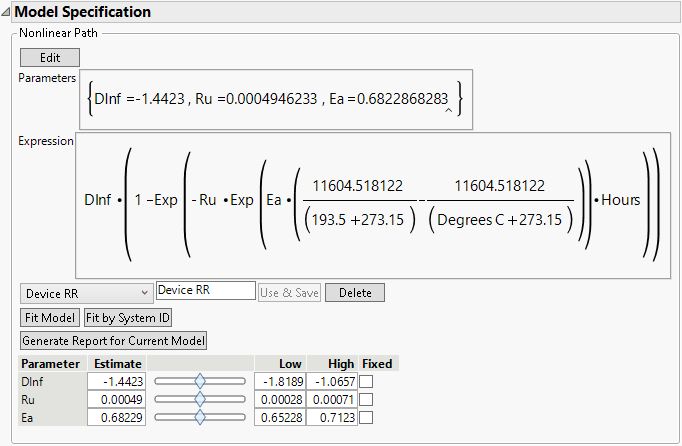The Fit Model button is used to fit the model to the data.

The Fit by System ID is used to fit the model to every level of Label, System ID.

The Delete button is used to delete a model from the model menu.

The Generate Report for Current Model button creates a report for the current model settings. See Model Reports.

The initial parameter values are shown at the bottom, along with sliders for visualizing how changes in the parameters affect the model. The fitted lines are shown on the Overlay plot. Move the parameter sliders to see how changes affect the fitted lines.

Here are the parameters for the Reaction Rate model (Meeker and Escobar 1998):

Dinf (D) - asymptotic degradation level

Ru (RU) - reaction rate at use temperature (tempU)

Ea (Ea) - reaction-specific activation energy

The above parameters are calculated as follows:

D(t; temp) = D x {1-exp[-RU x AF(temp) x t]}

where RU is the reaction rate at use temperature tempU, RU x AF(temp) is the reaction rate at a general temperature temp, and for temp > tempU, AF(temp) > 1

and

AF(temp) =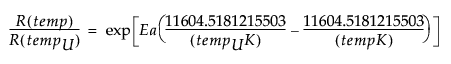where tempUK and tempK are temperatures expressed on the Kelvin scale.

To compute the optimal values for the parameters, click the Fit Model or Fit by System ID button.

To fix a value for a parameter, check the box under Fixed for the parameter. When fixed, that parameter is held constant in the model fitting process.

### Entering a Model with the Formula Editor

You can use the Formula Editor to enter a model. Click the Edit button to open the Formula Editor to enter parameters and the model. For more information about entering parameters and formulas in the Formula Editor, see Create Formulas in JMP in Using JMP.

Note: To view the Edit button displayed in Figure 7.12, you must select the interactive formula editor preference (File > Preferences > Platforms > Degradation > Use Interactive Formula Editor) before launching the Degradation platform.

Figure 7.12 Alternate Model Specification Report### Fit a Custom Model

If you want to fit a custom model, you must first create a formula column with initial parameter estimates. This method requires a few more steps than fitting a built-in model, but it allows any nonlinear model to be fit. Also, you can provide a custom loss function, and specify several other options for the fitting process.

1. Open your data table.

2. Create a new column in the data table.

3. Open the Formula Editor for the new column.

4. Select Parameters from the list in the lower left corner.

5. Click New Parameter.

6. Enter the name of the parameter.

7. Enter the initial value of the parameter.

Repeat steps 4 to 6 to create all the parameters in the model.

8. Build the model formula using the data table columns, parameters, and formula editor functions.

9. Click OK.

#### Parameters for Models with a Grouping Variable

In the formula editor, when you add a parameter, note the check box for Expand Into Categories, selecting column. This option is used to add several parameters (one for each level of a categorical variable for example) at once. When you select this option, a window appears that enables you to select a column. After selection, a new parameter appears in the Parameters list with the name D_column, where D is the name that you gave the parameter. When you use this parameter in the formula, a Match expression is inserted, containing a separate parameter for each level of the grouping variable.

### Model Library

The Model Library can assist you in creating a formula column with parameters and initial values. Click Model Library under Model Specification to open the library. Select a model in the list to see its formula in the Formula box.

Click Show Graph to show a 2-D theoretical curve for one-parameter models and a 3-D surface plot for two-parameter models. No graph is available for models with more than two explanatory (X) variables. Use the slider bars to change the default starting values for the parameters. You can also click the values and enter new values directly.

The Reset button sets the starting values for the parameters back to their default values.

Click Show Points to overlay the actual data points to the plot. A window opens, asking you to assign columns into X and Y roles, and an optional Group role. The Group role allows for fitting the model to every level of a categorical variable. If you specify a Group role here, also specify the same column in the Label, System ID role in the platform launch window.

For most models, the starting values are constants. Showing points enables you to adjust the parameter values to see how well the model fits for different values of the parameters.

Click Make Formula to create a new column in the data table. This column has the formula as a function of the specified X variable and uses the parameter values specified in the graph window.

Note: If you click Make Formula before you click the Show Graph or Show Points buttons, you are asked to provide the X and Y roles, and an optional Group role. After that, you are brought back to the plot so that you have the option to adjust the starting values for the parameters. Once the starting values for the parameters are satisfactory, click Make Formula again to create the new column.

Once the formula is created in the data table, click the Degradation Data Analysis red triangle and select Redo > Redo Analysis. Then, return to the column selection shown in Figure 7.10. Select the column that contains the model, and then click OK. You are returned to the Nonlinear Path Model Specification. For more information about all the features for that specification, see Model Specification Details.

Note: You can customize the models included in the Nonlinear Model Library by modifying the built-in script named NonlinLib.jsl. This script is located in the Resources/Builtins folder in the folder that contains JMP (Windows) or in the Application Package (macOS).

Want more information? Have questions? Get answers in the JMP User Community (community.jmp.com).